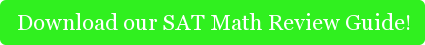#### What is the first thing that pops in your mind if you think about math? If you are anything like most people I know, you probably think about long equations and numbers. After all, that’s what math is, isn’t it?

Well, you are right and wrong at the same time (don’t you hate it when that happens?). Certainly, math does involve a lot of equations and numbers. But that’s not all there is to math. You certainly need to be comfortable with equations and numbers, but unfortunately, you need to be comfortable with much, much more. From years of working with many students, I have noticed a common trend. A lot of students will be very nimble  with arithmetic. However, when they read a word problem that gives them no equations they feel confused and frustrated: Why do textbooks have to do that? If you just give the same problem to students in an equation form, they would know how to solve it easily. Why go through this song and dance first?

Well, if you think about it, math is useful in everyday life. We do math daily, whether we realize it or not. When we go shopping, when we scale up or down a recipe, we are in fact working with equations and doing math. Now, ready for the shocking part? Almost never are the problems we face in real life given to us in an equation form. The problems we face are much like the word problems in the tests. We think about the real life scenario and in our heads translate it into an easy-to-work-with equation and solve it. So, the word problems in tests are much closer to what we actually have deal with in real life. Now, that’s actually more useful than practicing with abstract equations!

Now, a math test's problems are actually harder (sometimes, much harder), then the ones you face when you go shopping and have to estimate how much things cost. However, there is good news here. You can use the same methods you use daily to solve the harder math problems.

How do you do that? Start by reading the problem and taking notes on a piece of paper. In taking these notes, be careful to do it in a way that seems more clear to you. In some cases, this might involve a drawing.

Let’s run through an example taken from the Princeton Review Math Subject test. As a tutor for the math subject test, I know that this kind of problems often stumps students, but it really doesn't need to if you keep it simple:

Nicoletta deposits \$150 dollars in her savings account. If this deposit represents a 12 percent increase in Nicoletta’s savings, then how much does her saving account contain after the deposit?

Let x=Nicoletta's savings.

So 0.12x=150. So, x must be 150/0.12=\$1250.

This tells me Nicoletta had \$1250 in her account. Is this the answer?

This is where it’s important to go back and read the question carefully. What do they want you to figure out? How much was in her account? No, they are asking how much does her saving account contain after the deposit?

So, if the account had \$1250 and she added \$150, she now has \$1400. And that is the correct answer.

By drawing and rewriting the problem in a more clear way, it was easy to figure out the correct answer. Also, crucial to this problem, and to many other problems, was to carefully read the question and really understand what was being asked of you. I can’t emphasize how important this last part is. You always need to be crystal clear on what you are supposed to find out. You might solve an equation and get the correct answer, but always make sure that it is to the right question. Here, you needed to find out how much money Nicoletta had after the deposit, not before it.

The general principle described here applied to many math problems. Once you have translated the words into an equation, the hard part is over.